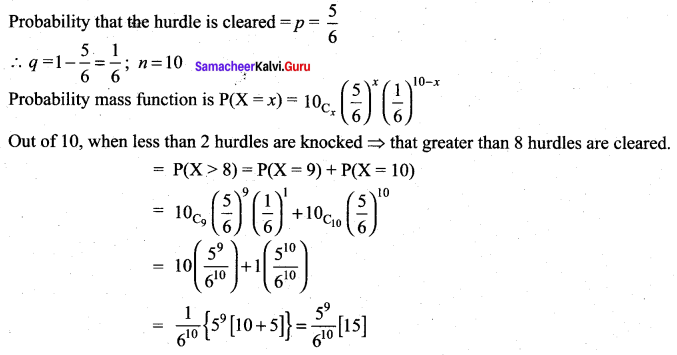## Tamilnadu Samacheer Kalvi 12th Maths Solutions Chapter 11 Probability Distributions Ex 11.5

Question 1.
Compute P(X = k) for the binomial distribution, B(n, p) where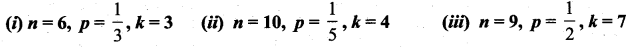Solution: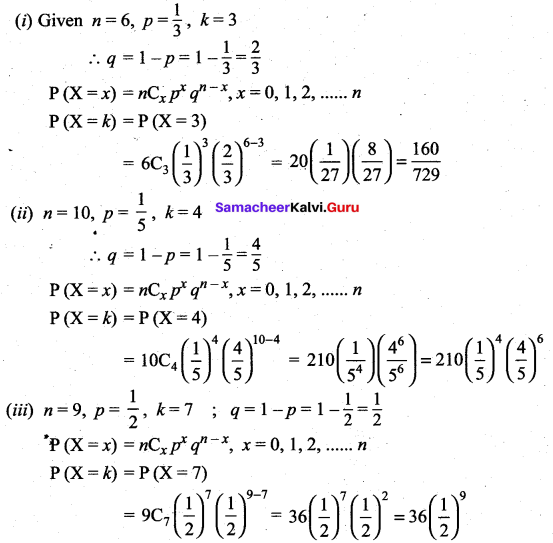Question 2.
The probability that Mr. Q hits a target at any trial is $$\frac{1}{4}$$. Suppose he tries at the target 10 times. Find the probability that he hits the target
(i) exactly 4 times
(ii) at least one time.
Solution:
Let ‘p’ be the probability of hitting the trial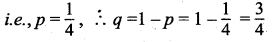and number of trials ‘n’ = 10
Probability of ‘x’ success in ‘n’ trials is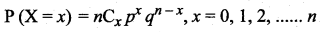(i) Probability that Mr.Q hits the target exactly 4 times is(ii) Probability that Mr.Q hits the target atleast one time is
P(X ≥ 1) = 1 – P(X < 1)
= 1 – P(X = 0)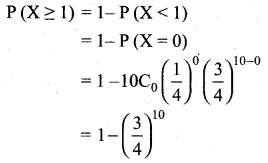Question 3.
Using binomial distribution find the mean and variance of X for the following experiments
(i) A fair coin is tossed 100 times, and X denote the number of heads.
(ii) A fair die is tossed 240 times, and X denote the number of times that four appeared.
Solution:
(i) n = 100, ‘X’ denotes the number of heads.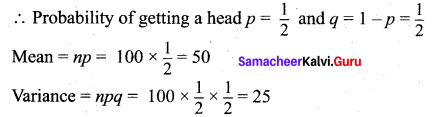(ii) n = 240, ‘X’ denotes the number of times four appeared.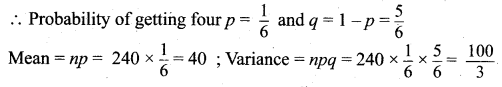Question 4.
The probability that a certain kind of component will survive a electrical test is $$\frac{3}{4}$$. Find the probability that exactly 3 of the 5 components tested survive.
Solution:
Given n = 5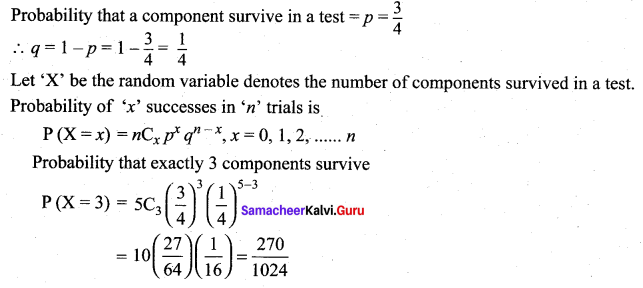Question 5.
A retailer purchases a certain kind of electronic device from a manufacturer. The manufacturer indicates that the defective rate of the device is 5%. The inspector of the retailer randomly picks 10 items from a shipment. What is the probability that there will be
(i) at least one defective item
(ii) exactly two defective items.
Solution:
Given n = 10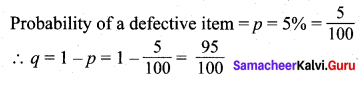Let ‘X’ be the random variable denotes the number of defective items.
∴ Probability of ‘x’ successes in ‘n’ trials is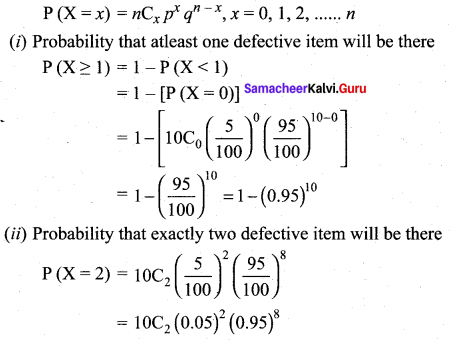Question 6.
If the probability that a fluorescent light has a useful life of at least 600 hours is 0.9, find the probabilities that among 12 such lights.
(i) exactly 10 will have a useful life of at least 600 hours;
(ii) at least 11 will have a useful life of at least 600 hours;
(iii) at least 2 will not have a useful life of at least 600 hours.
Solution:
Given n = 12
Probability that a fluorescent light has a life of atleast of 600 hours is p = 0.9
∴ q = 1 – p = 1 – 0.9 = 0.1
Let ‘X’ be the number of bulbs.
∴ Probability of ‘x’ successes in ‘n’ trials is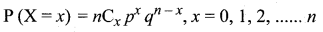(i) Probability that exactly 10 bulbs will have a useful life of atleast 600 hours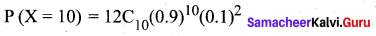(ii) Probability that atleast 11 will have a useful life of atleast 600 hours is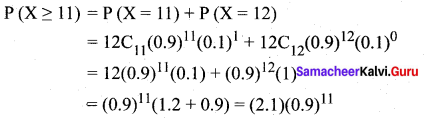(iii) Probability that atleast 2 will not have a useful life of 600 hours is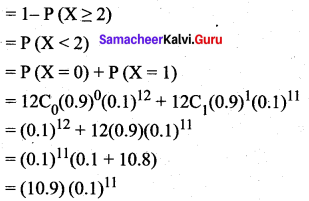Question 7.
The mean and standard deviation of a binomial variate X are respectively 6 and 2. Find
(i) the probability mass function
(ii) P(X = 3)
(iii) P(X ≥ 2) .
Solution: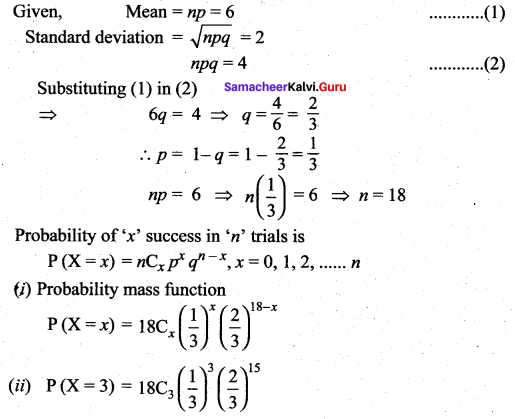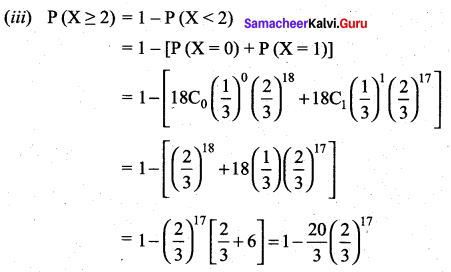Question 8.
If X ~ B(n, p) such that 4P(X = 4) = P(x = 2) and n = 6. Find the distribution, mean and standard deviation.
Solution: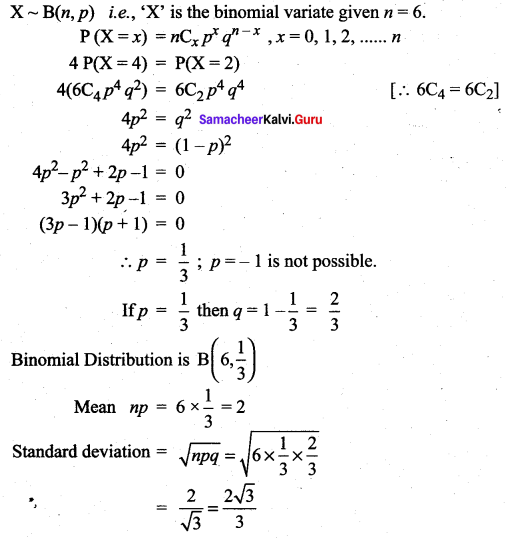Question 9.
In a binomial distribution consisting of 5 independent trials, the probability of 1 and 2 successes are 0.4096 and 0.2048 respectively. Find the mean and variance of the distribution.
solution:
Number of trials n = 5
Probability of ‘x’ successes in ‘n’ trials is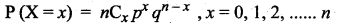Given P(X = 1) = 0.4096 and P (X = 2) = 0.2048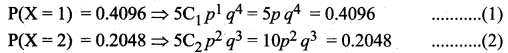Dividing Eq.(1) by Eq.(2)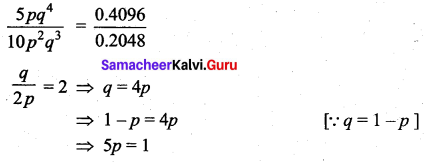### Samacheer Kalvi 12th Maths Solutions Chapter 11 Probability Distributions Ex 11.5 Additional Problems

Question 1.
In a Binomial distribution if n = 5 and P(X = 3) = 2P(X = 2) find p.
Solution: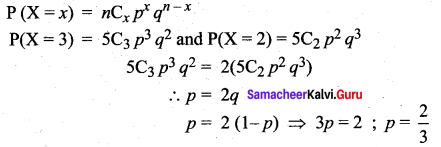Question 2.
If the sum of mean and variance of a Binomial Distribution is 4.8 for 5 trials find the distribution.
Solution:
np + npq = 4.8 ⇒ np (1 + q) = 4.8
5p [1 + (1 – p)] = 4.8
p2 – 2p + 0.96 = 0 ⇒ p = 1.2, 0.8
∴ p = 0.8 ; q = 0.2 [∵ p cannot be greater than 1]
∴ The Binomial distribution is P[X = x] = 5Cx (0.8)x (0.2)5 – x, x = 0 to 5

Question 3.
If on an average 1 ship out of 10 do not arrive safely to ports. Find the mean and the standard deviation of the ships returning safely out of a total of 500 ships.
Solution:
Probability of a ship arriving safely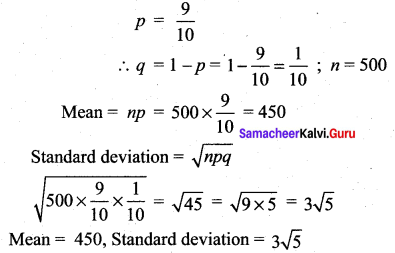Question 4.
The overall percentage of passes in a certain examination is 80. If six candidates appear in the examination what is the probability that at least five pass the examination.
Solution:
Pass percentage = 80%
∴ Probability of a candidate passing in the examination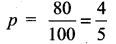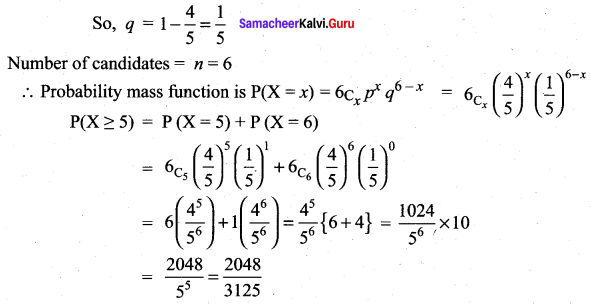Question 5.
In a hurdle race a player has to cross 10 hurdles. The probability that he will clear each hurdle is 5/6. What is the probability that he will knock down less than 2 hurdles?
Solution: